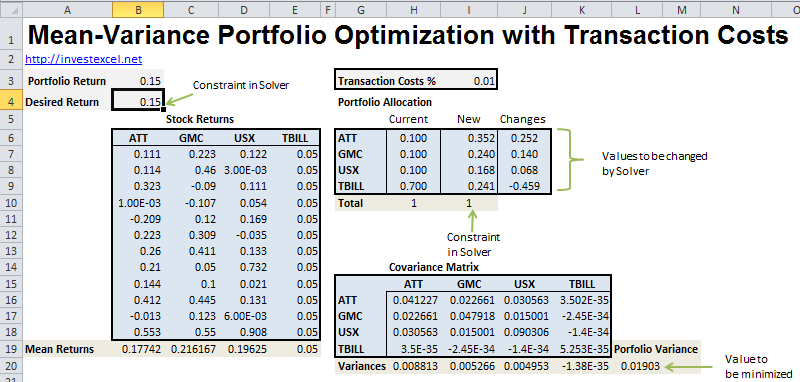# Mean-Variance Optimization with Transaction Costs

This Excel spreadsheet demonstrates the classic mean-variance approach for portfolio optimization, but includes the added complication of transaction costs.

Equities often have different characteristics.  For example, some are more volatile but offer a potentially greater return, while other equities increase in value when the wider market goes down.

By carefully constructing a portfolio with equities with different characteristics, risk is minimized while maintaining a superior return.

This problem was mathematically formalized by Markowitz in the 1950s (for which he won a Nobel prize some forty years later). His pioneering work  is a cornerstone of modern financial theory, and is known as mean-variance optimization. It is relatively simple to understand – we specify a desired return, and then pick investment weights which minimizes risk (represented by the variance of the returns).

For anything beyond the most trivial of problems, mean-variance optimization requires numerical optimizers.  Excel is a popular tool for this because it is ubiquitous and its advanced optimizers can solve complex problems.  This spreadsheet, for example, implements mean-variance optimization to determine the best investment weights in a portfolio of three stocks and one risk-free asset.

The spreadsheet presented in this article, however, includes the effect of transaction costs on mean-variance optimization.  Transaction costs can often be a significant issue when re-balancing an existing portfolio

The spreadsheet finds the change required to re-balance a portfolio (so that it lies on the efficient frontier), given transaction costs of 1% on every 1% change in the existing investment weights.

The transaction costs effectively reduce the expected return of the portfolio as follows:  Portfolio Return = Expected Return – Transaction Costs * (fraction of the portfolio bought and sold).

The relatively simple method used to determine transaction costs can easily be altered to model real-life behavior.

The spreadsheet is easy to use.  Full instructions are given in the spreadsheet itself, and an outline is given in the screengrab below.  The optimization process demands the use of Excel’s Solver functionality (the required settings are already given in the spreadsheet).This paper offers a  more complete guide to mean-variance optimization with transaction costs.

Other portfolio optimization approaches are also available. For example, you can maximize the Omega Ratio of a portfolio to determine the optimum investment weights.